# Search by Topic

#### Resources tagged with Addition & subtraction similar to What Do You Need?:

Filter by: Content type:
Age range:
Challenge level:

### There are 211 results

Broad Topics > Calculations and Numerical Methods > Addition & subtraction### As Easy as 1,2,3

##### Age 11 to 14 Challenge Level:

When I type a sequence of letters my calculator gives the product of all the numbers in the corresponding memories. What numbers should I store so that when I type 'ONE' it returns 1, and when I type. . . .### Zios and Zepts

##### Age 7 to 11 Challenge Level:

On the planet Vuv there are two sorts of creatures. The Zios have 3 legs and the Zepts have 7 legs. The great planetary explorer Nico counted 52 legs. How many Zios and how many Zepts were there?### Chameleons

##### Age 11 to 14 Challenge Level:

Whenever two chameleons of different colours meet they change colour to the third colour. Describe the shortest sequence of meetings in which all the chameleons change to green if you start with 12. . . .### Multiply the Addition Square

##### Age 11 to 14 Challenge Level:

If you take a three by three square on a 1-10 addition square and multiply the diagonally opposite numbers together, what is the difference between these products. Why?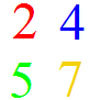### And So on and So On

##### Age 11 to 14 Challenge Level:

If you wrote all the possible four digit numbers made by using each of the digits 2, 4, 5, 7 once, what would they add up to?### Adding and Subtracting Positive and Negative Numbers

##### Age 11 to 14

How can we help students make sense of addition and subtraction of negative numbers?### Number Tracks

##### Age 7 to 11 Challenge Level:

Ben’s class were cutting up number tracks. First they cut them into twos and added up the numbers on each piece. What patterns could they see?### Largest Number

##### Age 11 to 14 Challenge Level:

What is the largest number you can make using the three digits 2, 3 and 4 in any way you like, using any operations you like? You can only use each digit once.### Digit Sum

##### Age 11 to 14 Challenge Level:

What is the sum of all the digits in all the integers from one to one million?### Score

##### Age 11 to 14 Challenge Level:

There are exactly 3 ways to add 4 odd numbers to get 10. Find all the ways of adding 8 odd numbers to get 20. To be sure of getting all the solutions you will need to be systematic. What about. . . .### Making Sense of Positives and Negatives

##### Age 11 to 14

This article suggests some ways of making sense of calculations involving positive and negative numbers.### Being Resilient - Primary Number

##### Age 5 to 11 Challenge Level:

Number problems at primary level that may require resilience.### 3388

##### Age 11 to 14 Challenge Level:

Using some or all of the operations of addition, subtraction, multiplication and division and using the digits 3, 3, 8 and 8 each once and only once make an expression equal to 24.### Arrange the Digits

##### Age 11 to 14 Challenge Level:

Can you arrange the digits 1,2,3,4,5,6,7,8,9 into three 3-digit numbers such that their total is close to 1500?### Jugs of Wine

##### Age 11 to 14 Challenge Level:

You have four jugs of 9, 7, 4 and 2 litres capacity. The 9 litre jug is full of wine, the others are empty. Can you divide the wine into three equal quantities?### Subtraction Surprise

##### Age 7 to 14 Challenge Level:

Try out some calculations. Are you surprised by the results?### Tis Unique

##### Age 11 to 14 Challenge Level:

This addition sum uses all ten digits 0, 1, 2...9 exactly once. Find the sum and show that the one you give is the only possibility.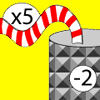### Function Machines

##### Age 7 to 11 Challenge Level:

If the numbers 5, 7 and 4 go into this function machine, what numbers will come out?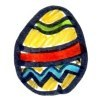### How Many Eggs?

##### Age 7 to 11 Challenge Level:

Peter, Melanie, Amil and Jack received a total of 38 chocolate eggs. Use the information to work out how many eggs each person had.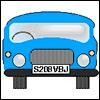### Numbered Cars

##### Age 7 to 11 Challenge Level:

I was looking at the number plate of a car parked outside. Using my special code S208VBJ adds to 65. Can you crack my code and use it to find out what both of these number plates add up to?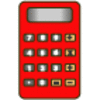### Clever Keys

##### Age 7 to 11 Short Challenge Level:

On a calculator, make 15 by using only the 2 key and any of the four operations keys. How many ways can you find to do it?### Kids

##### Age 11 to 14 Challenge Level:

Find the numbers in this sum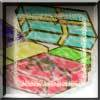##### Age 7 to 11 Challenge Level:

Use the information to work out how many gifts there are in each pile.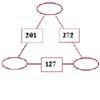### Number Juggle

##### Age 7 to 11 Challenge Level:

Fill in the missing numbers so that adding each pair of corner numbers gives you the number between them (in the box).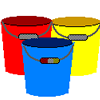### Buckets of Thinking

##### Age 7 to 11 Challenge Level:

There are three buckets each of which holds a maximum of 5 litres. Use the clues to work out how much liquid there is in each bucket.### Calendar Calculations

##### Age 7 to 11 Challenge Level:

Try adding together the dates of all the days in one week. Now multiply the first date by 7 and add 21. Can you explain what happens?### Abundant Numbers

##### Age 7 to 11 Challenge Level:

48 is called an abundant number because it is less than the sum of its factors (without itself). Can you find some more abundant numbers?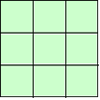### 1, 2, 3 Magic Square

##### Age 7 to 11 Challenge Level:

Arrange three 1s, three 2s and three 3s in this square so that every row, column and diagonal adds to the same total.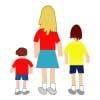### Sisters and Brothers

##### Age 7 to 11 Challenge Level:

Cassandra, David and Lachlan are brothers and sisters. They range in age between 1 year and 14 years. Can you figure out their exact ages from the clues?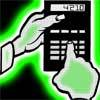### Reverse Trick

##### Age 7 to 11 Challenge Level:

Tell your friends that you have a strange calculator that turns numbers backwards. What secret number do you have to enter to make 141 414 turn around?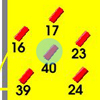### Throw a 100

##### Age 7 to 11 Challenge Level:

Can you score 100 by throwing rings on this board? Is there more than way to do it?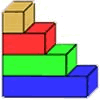### Rod Measures

##### Age 7 to 11 Challenge Level:

Using 3 rods of integer lengths, none longer than 10 units and not using any rod more than once, you can measure all the lengths in whole units from 1 to 10 units. How many ways can you do this?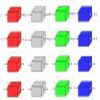### Machines

##### Age 7 to 11 Challenge Level:

What is happening at each box in these machines?### The Clockmaker's Birthday Cake

##### Age 7 to 11 Challenge Level:

The clockmaker's wife cut up his birthday cake to look like a clock face. Can you work out who received each piece?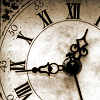### Clock Face

##### Age 7 to 11 Challenge Level:

Where can you draw a line on a clock face so that the numbers on both sides have the same total?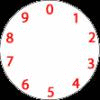### Dividing a Cake

##### Age 7 to 11 Challenge Level:

Annie cut this numbered cake into 3 pieces with 3 cuts so that the numbers on each piece added to the same total. Where were the cuts and what fraction of the whole cake was each piece?### Eleven

##### Age 11 to 14 Challenge Level:

Replace each letter with a digit to make this addition correct.### The Deca Tree

##### Age 7 to 11 Challenge Level:

Find out what a Deca Tree is and then work out how many leaves there will be after the woodcutter has cut off a trunk, a branch, a twig and a leaf.### Napier's Bones

##### Age 7 to 11 Challenge Level:

The Scot, John Napier, invented these strips about 400 years ago to help calculate multiplication and division. Can you work out how to use Napier's bones to find the answer to these multiplications?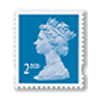### Penta Post

##### Age 7 to 11 Challenge Level:

Here are the prices for 1st and 2nd class mail within the UK. You have an unlimited number of each of these stamps. Which stamps would you need to post a parcel weighing 825g?### Scoring with Dice

##### Age 7 to 11 Challenge Level:

I throw three dice and get 5, 3 and 2. Add the scores on the three dice. What do you get? Now multiply the scores. What do you notice?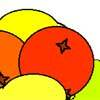### Oranges and Lemons

##### Age 7 to 11 Challenge Level:

On the table there is a pile of oranges and lemons that weighs exactly one kilogram. Using the information, can you work out how many lemons there are?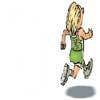### Rocco's Race

##### Age 7 to 11 Short Challenge Level:

Rocco ran in a 200 m race for his class. Use the information to find out how many runners there were in the race and what Rocco's finishing position was.### Pairs

##### Age 11 to 14 Challenge Level:

Ann thought of 5 numbers and told Bob all the sums that could be made by adding the numbers in pairs. The list of sums is 6, 7, 8, 8, 9, 9, 10,10, 11, 12. Help Bob to find out which numbers Ann was. . . .### Being Collaborative - Primary Number

##### Age 5 to 11 Challenge Level:

Number problems at primary level to work on with others.##### Age 5 to 11 Challenge Level:

Try out this number trick. What happens with different starting numbers? What do you notice?### Oddly

##### Age 7 to 11 Challenge Level:

Find the sum of all three-digit numbers each of whose digits is odd.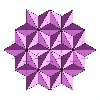### Next Number

##### Age 7 to 11 Short Challenge Level:

Find the next number in this pattern: 3, 7, 19, 55 ...### I'm Eight

##### Age 5 to 11 Challenge Level:

Find a great variety of ways of asking questions which make 8.### Got it for Two

##### Age 7 to 14 Challenge Level:

Got It game for an adult and child. How can you play so that you know you will always win?